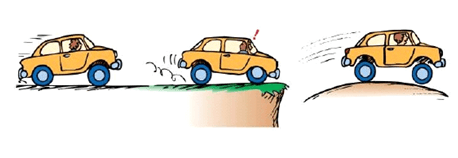Problems
230 - Acceleration (II)

Acceleration (II)

Time Limit: 1 sec

The Problem

We already know about Acceleration. The race is still going on. Now, we know the Velocity of the car increases uniformly from X ms-1 and after T s it becomes Y ms-1. Now he wanted to find the Acceleration and Distance of the car.The Input

The input file contains 3 numbers X, Y (-100 <=X, Y <=100) and T (0 <= T <= 200).

The Output

For each line of input output will show two values rounded to 1 decimal places for both Acceleration and Distance in two separated lines.

Sample Input

18.5  46.1  2.47

Sample Output

11.2

79.8

Problem Setter: Shaquib Mahmud

Special thanks to Samia Safa Ahmed